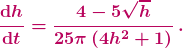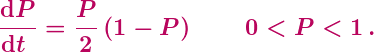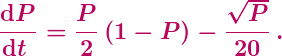## A Loss of Momentum

The VCE maths exams are over for another year. They were mostly uneventful, the familiar concoction of triviality, nonsense and weirdness, with the notable exception of the surprisingly good Methods Exam 1. At least two Specialist questions, however, deserve a specific slap and some discussion. (There may be other questions worth whacking: we never have the stomach to give VCE exams a close read.)

Question 6 on Specialist Exam 1 concerns a particle acted on by a force, and students are asked to

Find the change in momentum in kg ms-2 …

Doh!

The problem of course is that the suggested units are for force rather than momentum. This is a straight-out error and there’s not much to be said (though see below).

Then there’s Question 3 on part 2 of Specialist Exam 2. This question is concerned with a fountain, with water flowing in from a jet and flowing out at the bottom. The fountaining is distractingly irrelevant, reminiscent of a non-flying bee, but we have larger concerns.

In part (c)(i) of the question students are required to show that the height h of the water in the fountain is governed by the differential equationThe problem is with the final part (f) of the question, where students are asked

How far from the top of the fountain does the water level ultimately stabilise?

The question is typical in its clumsy and opaque wording. One could have asked more simply for the depth h of the water, which would at least have cleared the way for students to consider the true weirdness of the question: what is meant by “ultimately stabilise”?

The examiners are presumably expecting students to set dh/dt = 0, to obtain the constant, equilibrium solution (and then to subtract the equilibrium value from the height of the fountain because why not give students the opportunity to blow half their marks by misreading a convoluted question?) The first problem with that is, as we have pointed out before, equilibria of differential equations appear nowhere in the Specialist curriculum. The second problem is, as we have pointed out before, not all equilibria are stable.

It would be smart and good if the VCAA decided to include equilibrium solutions in the Specialist curriculum, along with some reasonable analysis and application. Until they do, however, questions such as the above are unfair and absurd, made all the more unfair and absurd by the inevitably awful wording.

********************

Now, what to make of these two questions? How much should VCAA be hammered?

We’re not so concerned about the momentum error. It is unfortunate, it would have confused many students and it shouldn’t have happened, but a typo is a typo, without deeper meaning.

It appears that Specialist teachers have been less forgiving, and fair enough: the VCAA examiners are notoriously nitpicky, sanctimonious and unapologetic, so they can hardly complain if the same, with greater justification, is done to them. (We also heard of some second-guessing, some suggestions that the units of “change in momentum” could be or are the same as the units of force. This has to be Stockholm syndrome.)

The fountain question is of much greater concern because it exemplifies systemic issues with the curriculum and the manner in which it is examined. Above all, assessment must be fair and reasonable, which means students and teachers must be clearly told what is examinable and how it may be examined. As it stands, that is simply not the case, for either Specialist or Methods.

Notably, however, we have heard of essentially no complaints from Specialist teachers regarding the fountain question; just one teacher pointed out the issue to us. Undoubtedly there were other teachers bothered by the question, but the relative silence in comparison to the vocal complaints on the momentum typo is stark. And unfortunate.

There is undoubted satisfaction in nitpicking the VCAA in a sauce for the goose manner. But a typo is a typo, and teachers shouldn’t engage in small-time point-scoring any more than VCAA examiners.

The real issue is that the current curriculum is shallow, aimless, clunky, calculator-poisoned, effectively undefined and effectively unexaminable. All of that matters infinitely more than one careless mistake.

## Update (24/02/19)

The exam Reports are now out, here and here. There’s no stupidity so large or so small that the VCAA won’t remain silent.

## The Madness of Crowd Models

Our fifth and penultimate post on the 2017 VCE exam madness concerns Question 3 of Section B on the Northern Hemisphere Specialist Mathematics Exam 2. The question begins with the logistic equation for the proportion P of a petri dish covered by bacteria:This is not a great start, since it’s a little peculiar using the logistic equation to model an area proportion, rather than a population or a population density. It’s also worth noting that the strict inequalities on P are unnecessary and rule out of consideration the equilibrium (constant) solutions P = 0 and P = 1.

Clunky framing aside, part (a) of Question 3 is pretty standard, requiring the solving of the above (separable) differential equation with initial condition P(0) = 1/2. So, a decent integration problem trivialised by the presence of the stupifying CAS machine. After which things go seriously off the rails.

The setting for part (b) of the question has a toxin added to the petri dish at time t = 1, with the bacterial growth then modelled by the equationWell, probably not. The effect of toxins is most simply modelled as depending linearly on P, and there seems to be no argument for the square root. Still, this kind of fantasy modelling is par for the VCAA‘s crazy course. Then, however, comes Question 3(b):

Find the limiting value of P, which is the maximum possible proportion of the Petri dish that can now be covered by the bacteria.

The question is a mess. And it’s wrong.

The Examiners’ “Report” (which is not a report at all, but merely a list of short answers) fails to indicate what students did or how well they did on this short, 2-mark question. Presumably the intent was for students to find the limit of P by finding the maximal equilibrium solution of the differential equation. So, setting dP/dt = 0 implies that the right hand side of the differential equation is also 0. The resulting equation is not particularly nice, a quartic equation for Q = √P. Just more silly CAS stuff, then, giving the largest solution P = 0.894 to the requested three decimal places.

In principle, applying that approach here is fine. There are, however, two major problems.

The first problem is with the wording of the question: “maximum possible proportion” simply does not mean maximal equilibrium solution, nor much of anything. The maximum possible proportion covered by the bacteria is P = 1. Alternatively, if we follow the examiners and needlessly exclude = 1 from consideration, then there is no maximum possible proportion, and P can just be arbitrarily close to 1. Either way, a large initial P will decay down to the maximal equilibrium solution.

One might argue that the examiners had in mind a continuation of part (a), so that the proportion begins below the equilibrium value and then rises towards it. That wouldn’t rescue the wording, however. The equilibrium solution is still not a maximum, since the equilibrium value is never actually attained. The expression the examiners are missing, and possibly may even have heard of, is least upper bound. That expression is too sophisticated to be used on a school exam, but whose problem is that? It’s the examiners who painted themselves into a corner.

The second issue is that it is not at all obvious – indeed it can easily fail to be true – that the maximal equilibrium solution for P will also be the limiting value of P. The garbled information within question (b) is instructing students to simply assume this. Well, ok, it’s their question. But why go to such lengths to impose a dubious and impossible-to-word assumption, rather than simply asking directly for an equilibrium solution?

To clarify the issues here, and to show why the examiners were pretty much doomed to make a mess of things, consider the following differential equation:By setting Q = √P, for example, it is easy to show that the equilibrium solutions are P = 0 and P = 1/4. Moreover, by considering the sign of dP/dt for P above and below the equilibrium P = 1/4, it is easy to obtain a qualitative sense of the general solutions to the differential equation:In particular, it is easy to see that the constant solution P = 1/4 is a semi-stable equilibrium: if P(0) is slightly below 1/4 then P(t) will decay to the stable equilibrium P = 0.

This type of analysis, which can readily be performed on the toxin equation above, is simple, natural and powerful. And, it seems, non-existent in Specialist Mathematics. The curriculum  contains nothing that suggests or promotes any such analysis, nor even a mention of equilibrium solutions. The same holds for the standard textbook, in which for, for example, the equation for Newton’s law of cooling is solved (clumsily), but there’s not a word of insight into the solutions.

And this explains why the examiners were doomed to fail. Yes, they almost stumbled into writing a good, mathematically rich exam question. The paper thin curriculum, however, wouldn’t permit it.